Home > CC2MN > Chapter cc29 > Lesson cc29.1.1 > Problem9-10

9-10.
1. Estimate the circumference or diameter for each of the following circles. For each one, give your reasoning. Homework Help ✎

1. The diameter is 1 cm. What is the circumference?

2. The diameter is 7.2 meters. What is the circumference?

3. The circumference is 1 mile. What is the diameter?

4. The circumference is 12 inches. What is the diameter?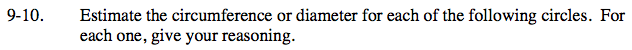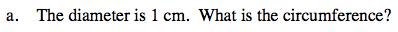The circumference of a circle can be thought of as the length of a string that wraps exactly around it once.
You can calculate the circumference by multiplying the diameter by π, which is just over 3.

The circumference is π cm, or just over 3 cm.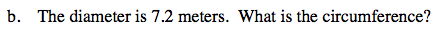What length is just over 3 times longer than 7.2 meters?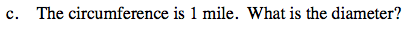Since the circumference is just over 3 times the diameter, it makes sense that the diameter is about one third the length of the circumference.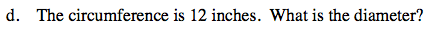Using the same hint as in part (c), approximate your answer by substituting 3 for π once again.

The diameter is approximately 4 inches.